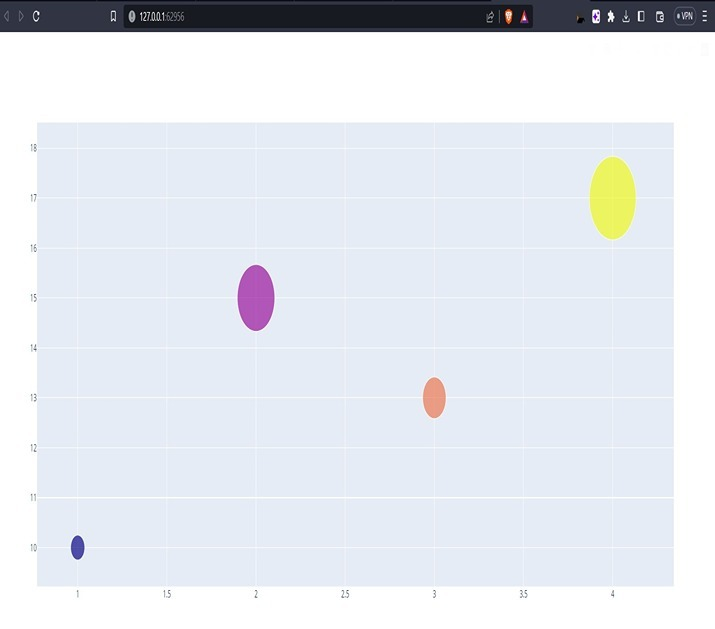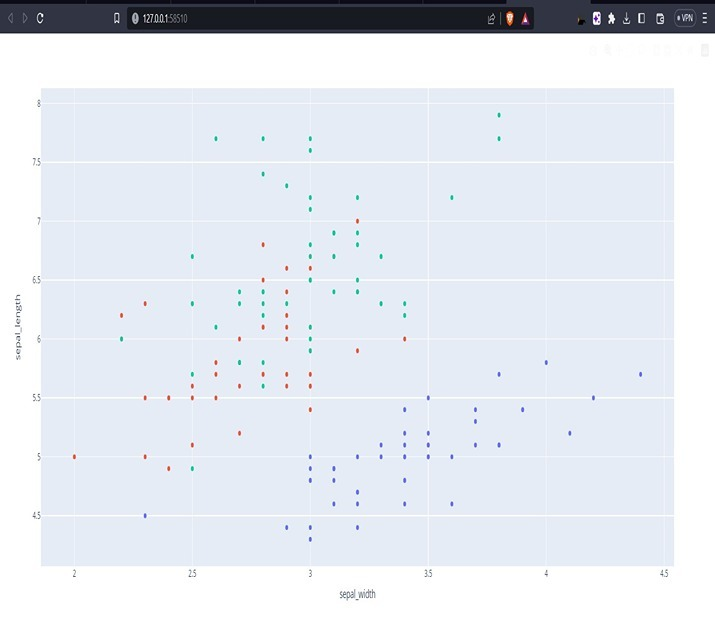# How to Hide Legend with Plotly Express and Plotly in Python?

Plotly is a strong Python library for making intelligent perceptions. It offers various highlights and customization choices to upgrade the appearance and usefulness of plots. One normal necessity in information perception is the capacity to stow away or eliminate the legend.The legend provides a key to interpret the different elements in a plot, such as colors or line styles. In this article, we will explore how to hide the legend using Plotly Express and Plotly, two popular libraries in Python for creating visualizations.

## Syntax

Before we dive into the different approaches to hide the legend in Plotly, let's first understand the syntax of the method we will be using in the following code examples −

fig.update_layout(showlegend=False)


The showlegend attribute is set to False to hide the legend in the plot.

## Algorithm

Here is a step-by-step algorithm to hide the legend in Plotly −

• Import the necessary libraries −

• import plotly.express as px for using Plotly Express.

• import plotly.graph_objects as go for using Plotly.

• Create a plot using either Plotly Express or Plotly −

• For Plotly Express − fig = px.<chart_type>(data_frame, ...)

• For Plotly − fig = go.Figure(data=[<trace(s)>], ...)

• Update the layout to hide the legend −

• fig.update_layout(showlegend=False)

• Display the plot −

• fig.show()

## Approach 1: Using Plotly Express

Plotly Express provides a simple and concise API for creating various types of plots. To hide the legend using Plotly Express, follow these steps −

### Example

#Import the necessary libraries
import plotly.express as px
#Create a scatter plot using Plotly Express
data = px.data.iris()
fig = px.scatter(data, x="sepal_width", y="sepal_length", color="species")
#Update the layout to hide the legend
fig.update_layout(showlegend=False)

#Display the plot

fig.show()


### Output### Explanation

Using Plotly Express -

Plotly Express provides a high-level interface for creating various types of plots with concise syntax. To hide the legend using Plotly Express, we follow a few simple steps.

First, we import the necessary library − import plotly.express as px. This allows us to utilize the functionalities of Plotly Express.

Next, we create a scatter plot using Plotly Express. For instance, let's assume we have a dataset containing measurements of sepal width, sepal length, and species of flowers. We can make the scatter plot with the accompanying code − fig = px.scatter(data, x="sepal_width", y="sepal_length", color="species"). This code determines the dataset, the x-pivot values (sepal width), the y-hub values (sepal length), and the varieties in view of the species.

Presently, we really want to refresh the format of the figure to conceal the legend.

We accomplish this by using the command − fig.update_layout(showlegend=False). This command sets the showlegend attribute to False, effectively hiding the legend.

Finally, we display the plot using fig.show(), which renders the scatter plot with the legend hidden. This approach offers a straightforward way to create interactive visualizations using Plotly Express while hiding the legend for a cleaner look.

## Approach 2: Using Plotly

Plotly provides more flexibility and control over the plot customization. To hide the legend using Plotly, follow these steps −

### Example

#Import the necessary libraries:
import plotly.graph_objects as go
#Create a scatter plot using Plotly:
data = go.Scatter(
x=[1, 2, 3, 4],
y=[10, 15, 13, 17],
mode='markers',
marker=dict(size=[30, 80, 50, 100], color=[0, 1, 2, 3])
)

fig = go.Figure(data=[data])
#Update the layout to hide the legend:
fig.update_layout(showlegend=False)
#Display the plot:
fig.show()


### Output### Explanation

Using Plotly

Plotly, unlike Plotly Express, provides more control and flexibility for customization. To hide the legend using Plotly, we follow a similar set of steps.

We begin by importing the necessary library − import plotly.graph_objects as go. This enables us to use the functionalities of Plotly.

Next, we create the scatter plot using Plotly. For instance, suppose we have a scatter plot with custom x and y values, as well as marker sizes and colors defined for each data point.

Now, similar to the previous approach, we update the layout to hide the legend by using fig.update_layout(showlegend=False). This command sets the showlegend attribute to False, ensuring that the legend is hidden.

Finally, we display the plot using fig.show(), which renders the scatter plot with the legend hidden. This approach provides greater flexibility in customizing the plot's appearance and behavior using Plotly's extensive features.

In summary, both approaches offer different levels of simplicity and customization. Plotly Express provides a concise and straightforward way to create visualizations with fewer lines of code, while Plotly allows for more control and flexibility in customizing the plot. By using the appropriate approach based on your requirements, you can easily hide the legend in Plotly visualizations and achieve a cleaner and more focused presentation of your data.

## Conclusion

In this article, we have learned how to hide the legend in Plotly using both Plotly Express and Plotly. By updating the layout of the figure and setting the showlegend attribute to False, we can easily remove the legend from our plots. This can be useful when the legend is not necessary or when we want to declutter the visualization. Remember that hiding the legend should be used thoughtfully, as it may impact the interpretability of the plot for the viewers. Experiment with these approaches and explore the vast capabilities of Plotly to create stunning and informative visual

Updated on: 27-Jul-2023

906 Views